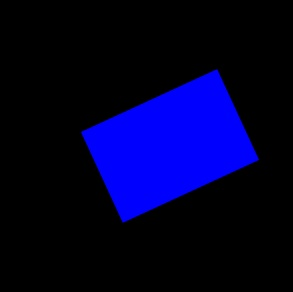## Rotate canvas on mouse drag

Get the source at Github

For a larger project of mine i needed to find a way to rotate cavas elements on mousedrag. So here’s my attempt to create this user interface functionality.

### Source and example

Watch the demo### Seperate steps

First I divided the script into logical steps to simplify the problem.

1. create a update function for the canvas.
2. setup the mousehandlers to register the “drag” event
3. create a helper function to calculate the angle between 2 coordinates

The key for this functionality is that the starting angle of the drag always differs. This causes the canvas drawing to jump to unwanted angles if the script does not compensate for this.

```\$( model.cnv ).mousedown(function(event){
// calculate click angle minus the last angle
var clickAngle = getAngle(cX + offX, cY + offY, event.clientX, event.clientY )
- model.angle;
\$( model.cnv ).bind("mousemove", clickAngle, function(event){
// calculate move angle minus the angle onclick
model.angle =  ( getAngle( cX + offX, cY + offY, event.clientX, event.clientY  )
- clickAngle);
updateRectangle(model.angle);
});
});
```

I have not tested the script with other shapes than rectangles and squares, but the script should work fine as long as the canvas elements have ‘bounding box’ with a width and height. Of course this example is a starting point and the interface could use some custom cursors or icons.